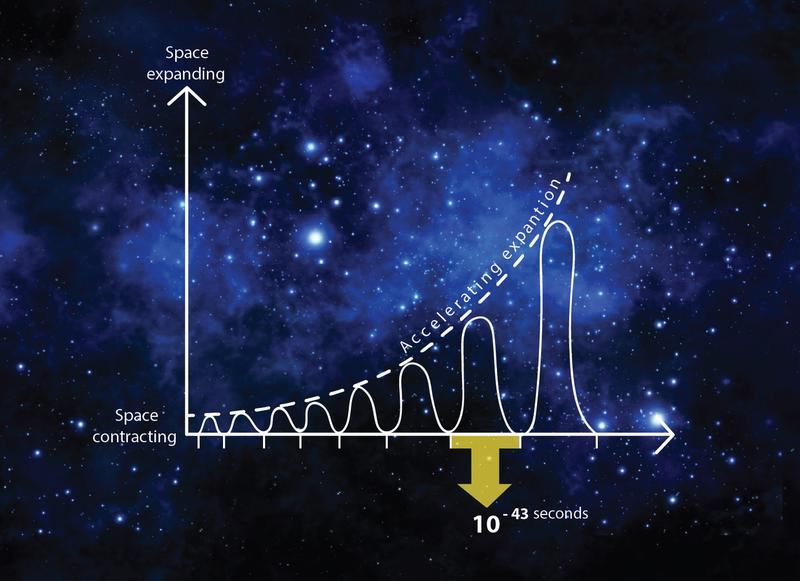# Something… out of nothing?How can something be created from nothingness? How is that a possibility? Furthermore, how can it be proven?

If we were to place a box under vacuum, theoretically, we would have a box of nothingness. But is it really empty? The answer is both ‘yes’ and ‘no’. Enter quantum fluctuation. A particle can be created in a vacuum, and disappear, provided that it happens very quickly.

In quantum physics, a quantum fluctuation is the temporary change in the amount of energy in a point in space, also known as the Heisenberg uncertainty principle. The uncertainty principle states that for a pair of conjugate variables such as position/momentum or energy/time, it is impossible to have a precisely determined value of each member of the pair at the same time.

Quantum physics and Special Theory of Relativity were further explained via Dirac’s famous equation.

Dirac equation(original)This equation describes particles and anti-particles. These particles are created in pairs, which can borrow energy from a vacuum before reuniting and cancelling each other out, thus returning the borrowed energy. These ‘virtual particles’ can appear and disappear trillions of times in a blink of an eye. One proof that this does happen, is by observing electrons of an atom in a vacuum. The electrons want to move on a flat plane around an atom. If the electrons bobble in their orbit around an atom, it is because of particles and anti-particles, interfering and affecting the electrons’ orbits.

Now, this all begs the question, ‘Why does any of this matter?’ Well, we know that the universe is expanding at an accelerated rate, but what is the driving force? One theory suggested that dark energy was the reason. A new theory puts forth the idea that, with the creation and destruction of these virtual particles, the universe oscillates between expansion and contraction. During these oscillations, the net effect is that the universe expands very slowly, but at an ever accelerating rate.

Translate »